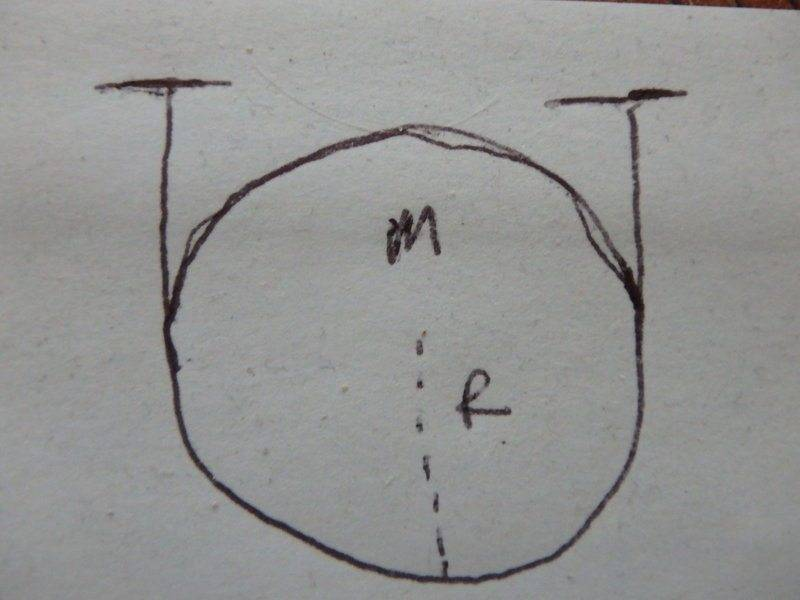# Frictionless Disk Supported by Massless String

hringsak
In the following diagram, a frictionless disc is supported by a massless string. This problem was given by the author of a book, and a solution was given to some questions that were asked about this diagram. One thing the author said in one of the solutions, was that the tension in the string was equal to Mg/2. Why is this? I would have thought that since the one string is supporting the entire mass of the disc that it should just be Mg.Nugatory
Mentor
One thing the author said in one of the solutions, was that the tension in the string was equal to Mg/2. Why is this? I would have thought that since the one string is supporting the entire mass of the disc that it should just be Mg.
Consider three setups:
1) The one in your diagram.
2) The one in your diagram, except that we've permanently fixed the string to the disk with a glob of glue at the lowest point.
3) Like #2, except that instead of one string passing through the glob of glue we have two strings both ending in the glob of glue and held onto the disk by the glue.

In #3 we clearly have two strings. Is the tension going to be the same or different in the three cases?

•hringsak
hringsak
Okay, that makes sense that each half of the string is supporting half of the mass. Instead of one end of the string being attached to the ceiling and one end to the disc, where the tension would be Mg, you have just half of the tension at each end. However, because in the midpoint of the string there is no glob of glue, which would provide a balancing force to both halves of the string as in setup #2, doesn't one half pull on the end of the other half, therefore making the tension Mg?

I do get your point that the glue in the middle in setup #2 is not even necessary, but isn't that because the tension from one half is balanced by tension from the other half?

hringsak
Wait, I get it now - because both ends are attached to the ceiling, they each are pulling with just half the force as in the example where just one end is attached to the ceiling. In that example you have the full force of Mg pulling in opposite directions. The fact that in the above diagram, both ends are attached to the ceiling, that results in just half the tension in the string.

CWatters